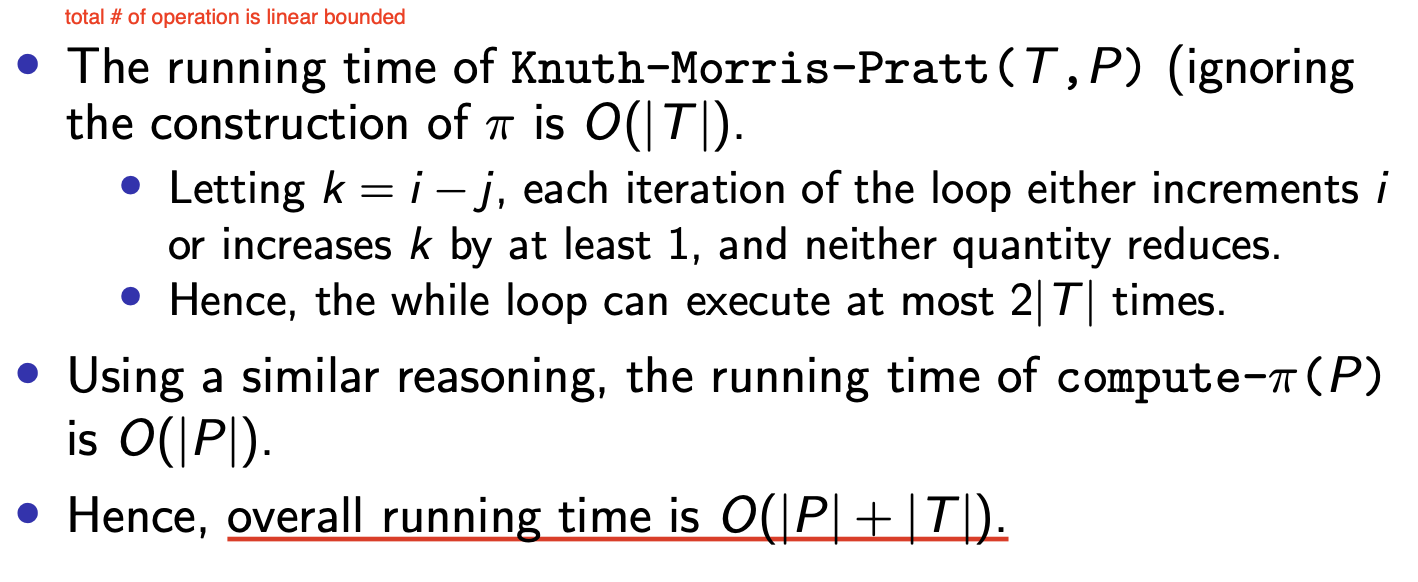# 高级算法设计:时间复杂度层级和字符串查找问题

## Fast, Slow and String Searching

Posted by R1NG on October 6, 2022 Viewed Times

# 时间复杂度层级和字符串查找算法

!!注: 完整详细的复习内容请参阅 《COMP36111 期末总复习》.

## Fast and Slow: 时间复杂度层级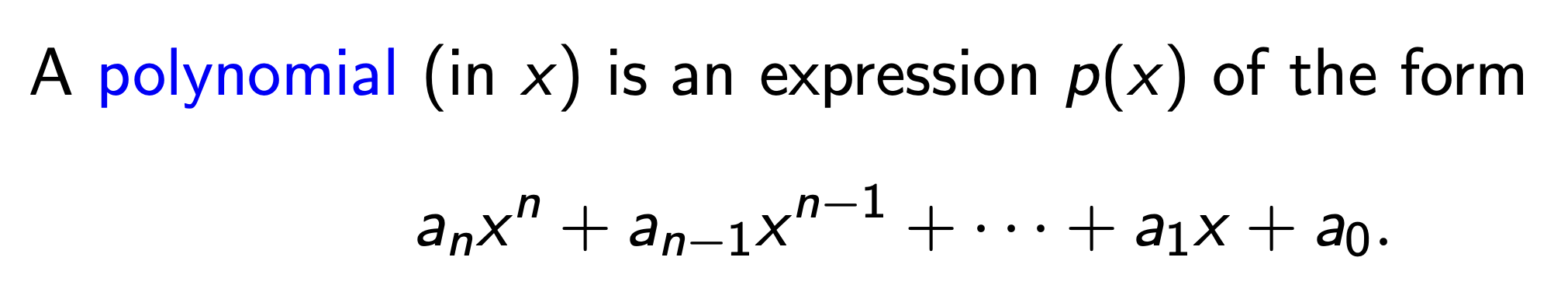$\forall n, f(n) \leqslant p(n)$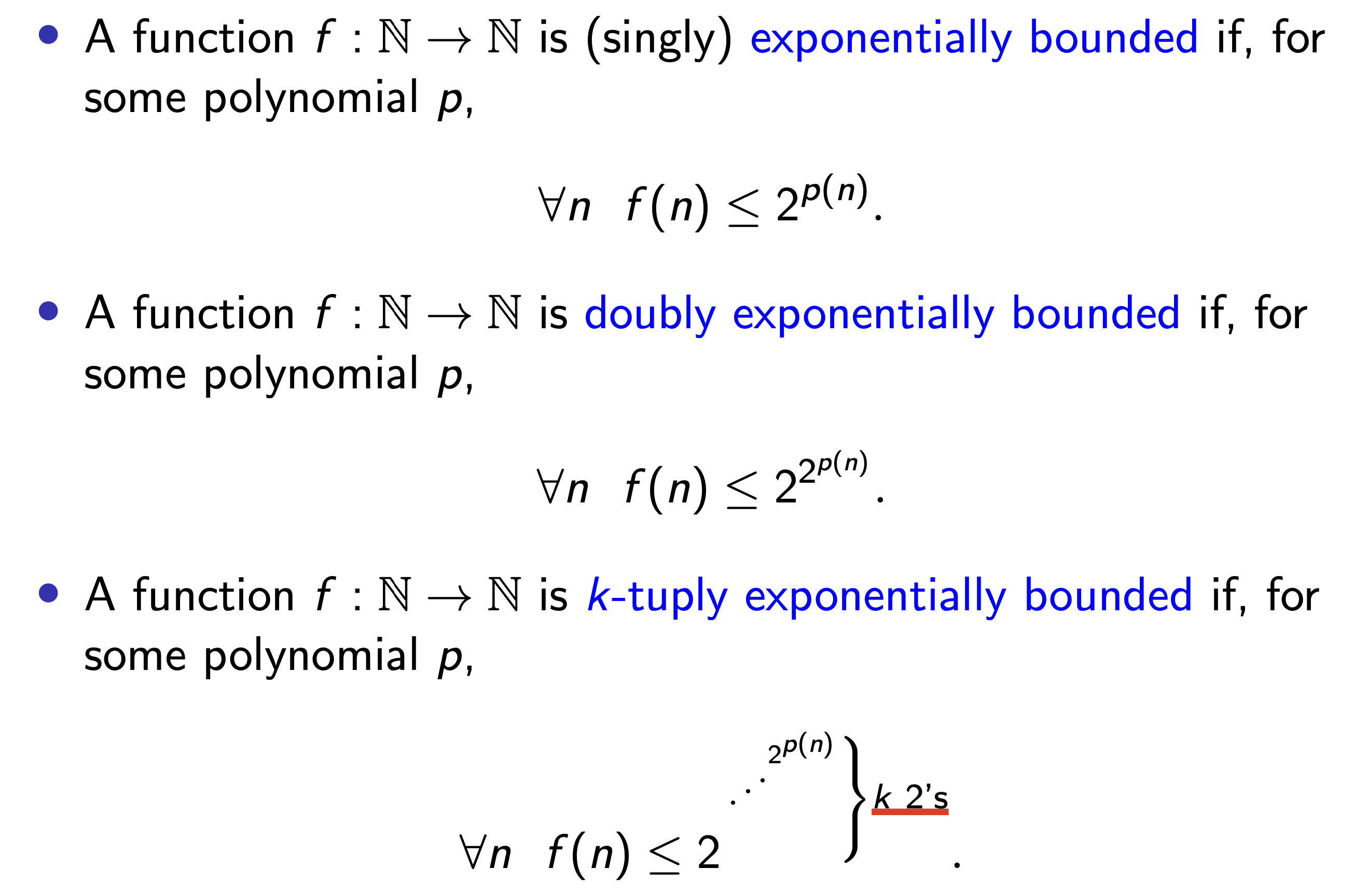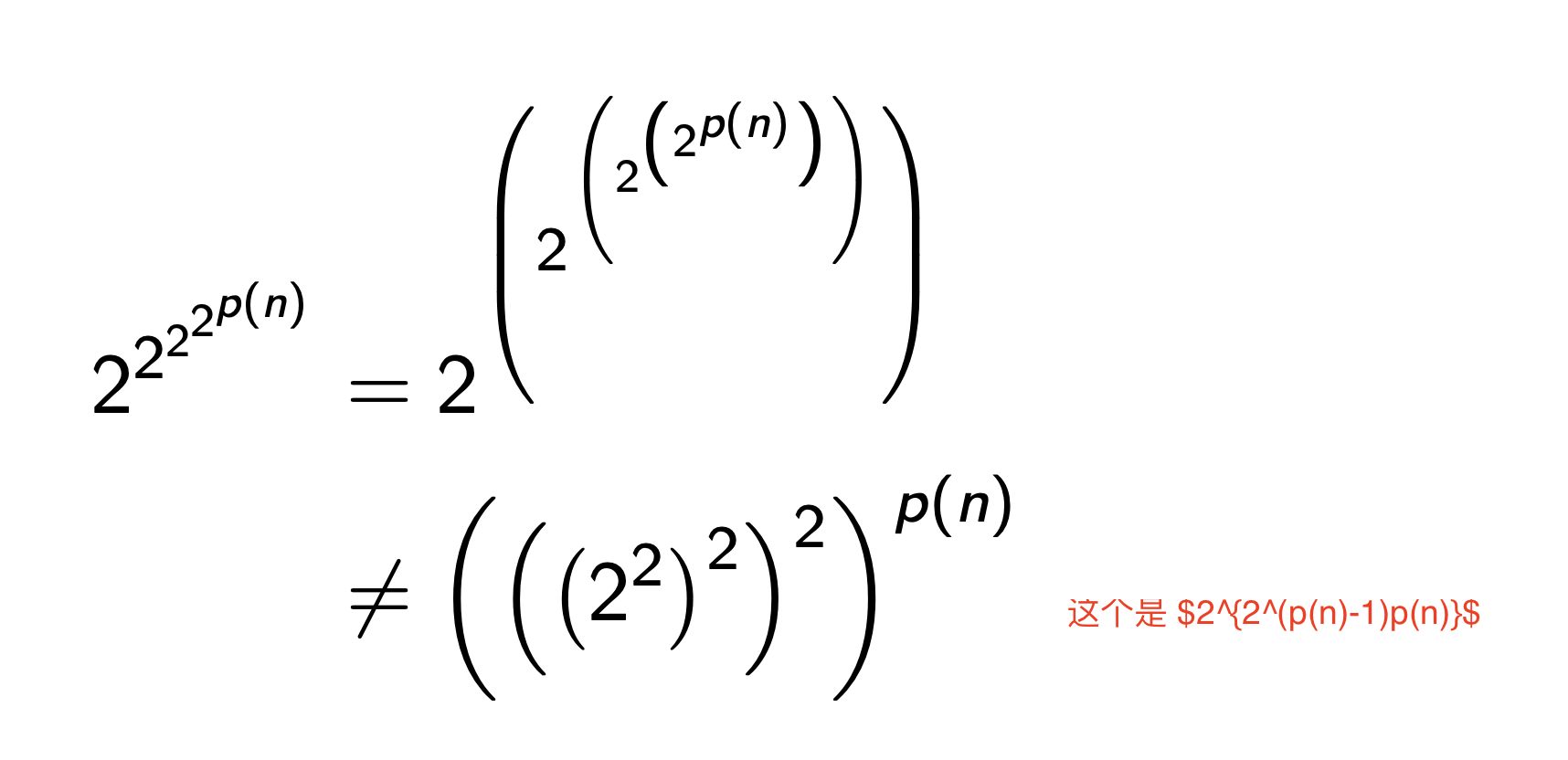1. $2^n$ 不是多项式有界 的, 但它是 一次指数有界 的.
2. $2^{n^2}$ 是 单指数有界 的.
3. $2^{2^n}$ 不是 一次指数有界 的, 但它是 二次指数有界 的.
4. 对于 $n!$, 只要 $n$ 足够大 (大于 $2$), 则它是 一次指数有界 的: $2^n < n! = 2^{n\log{n}} < 2^{n^2}$.

### 塔函数 (Tower | Tetration Function):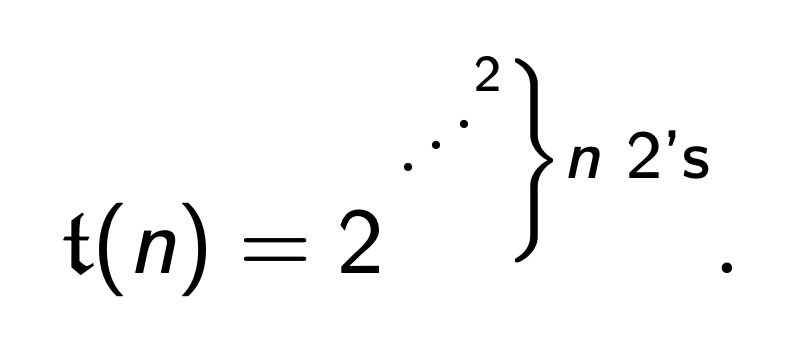LOOP 语言中我们只考虑下列所示的语法: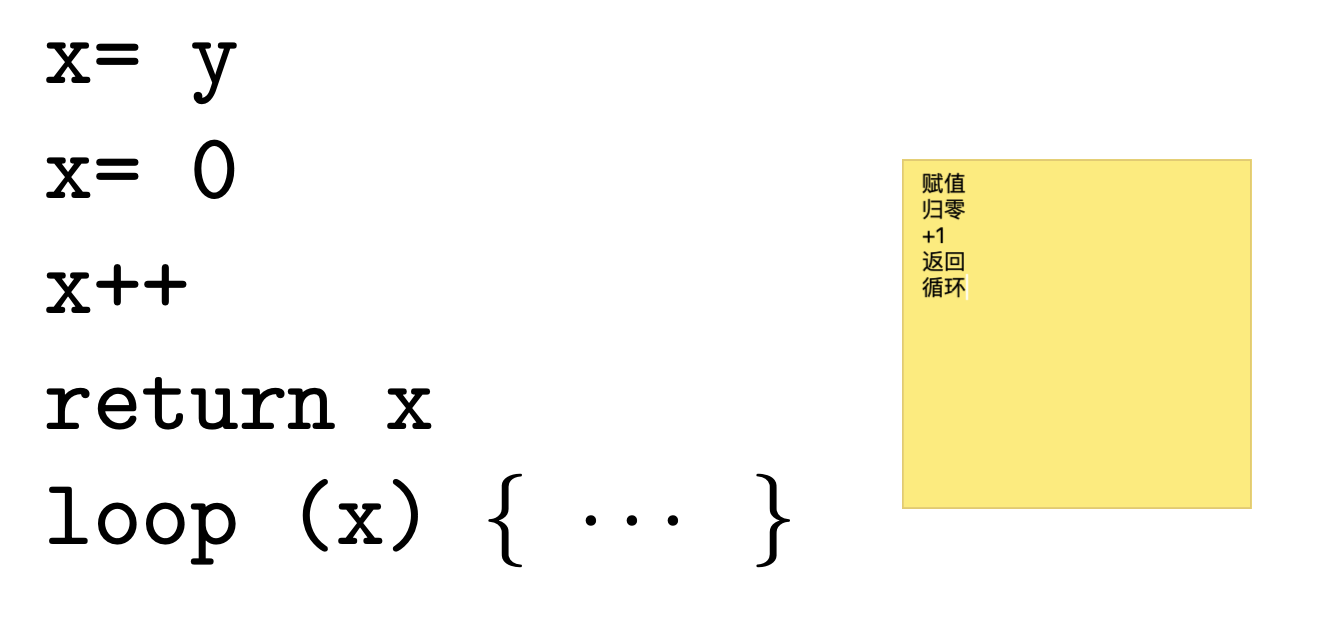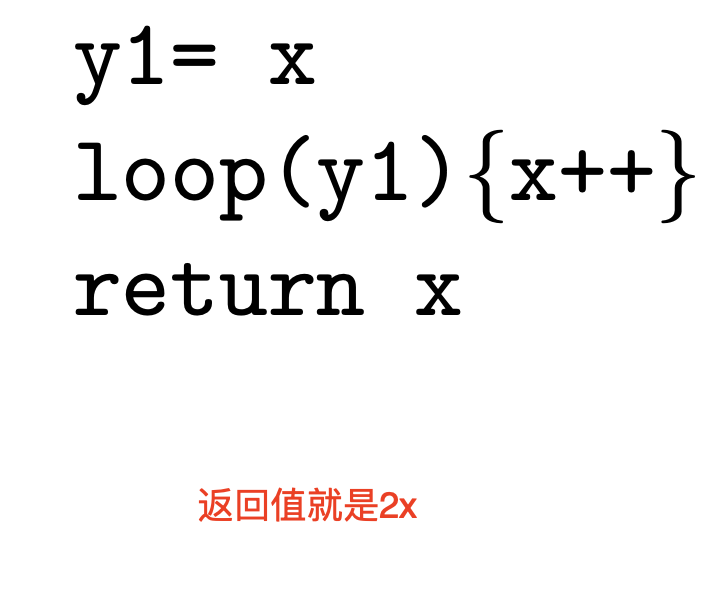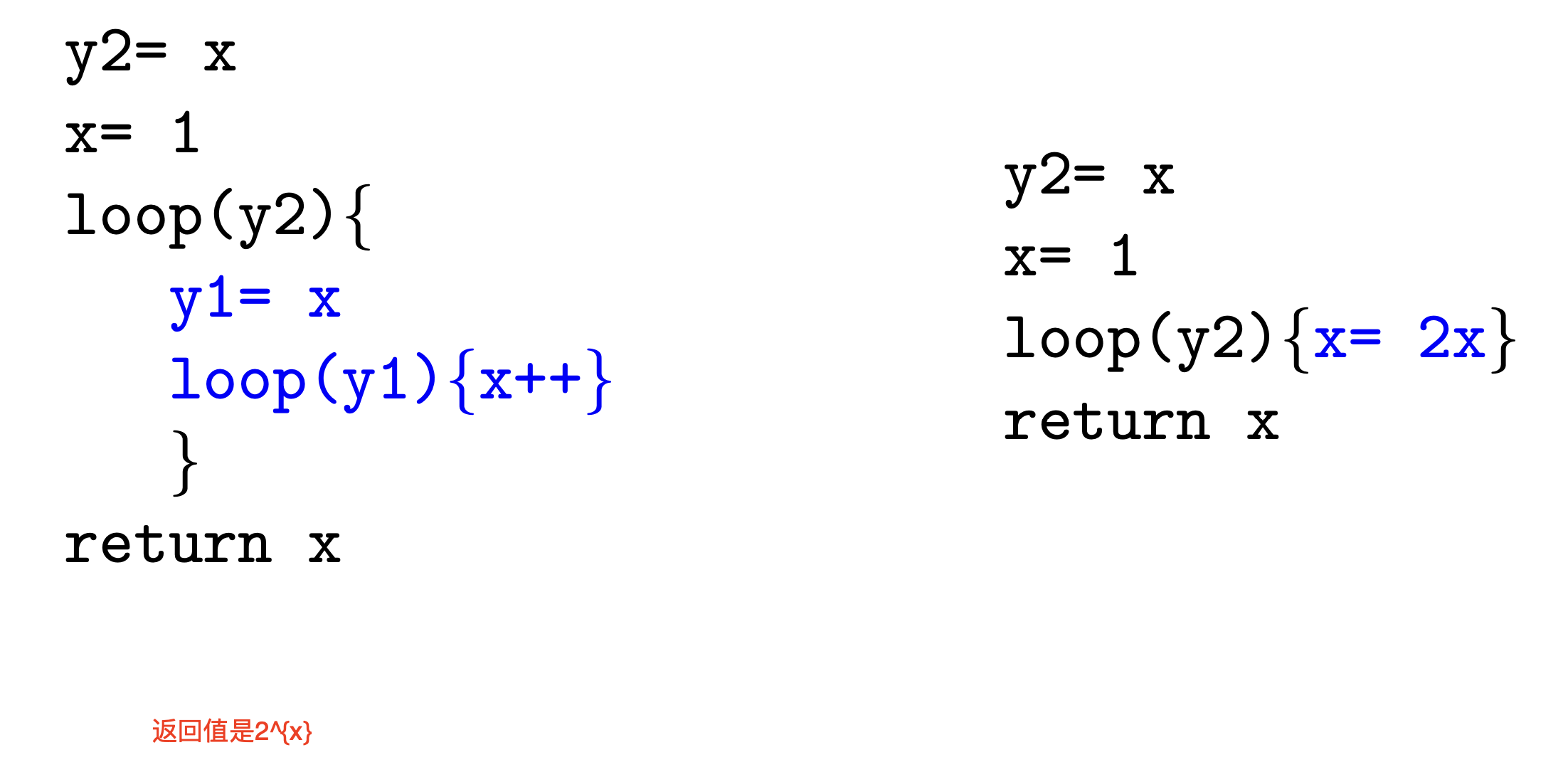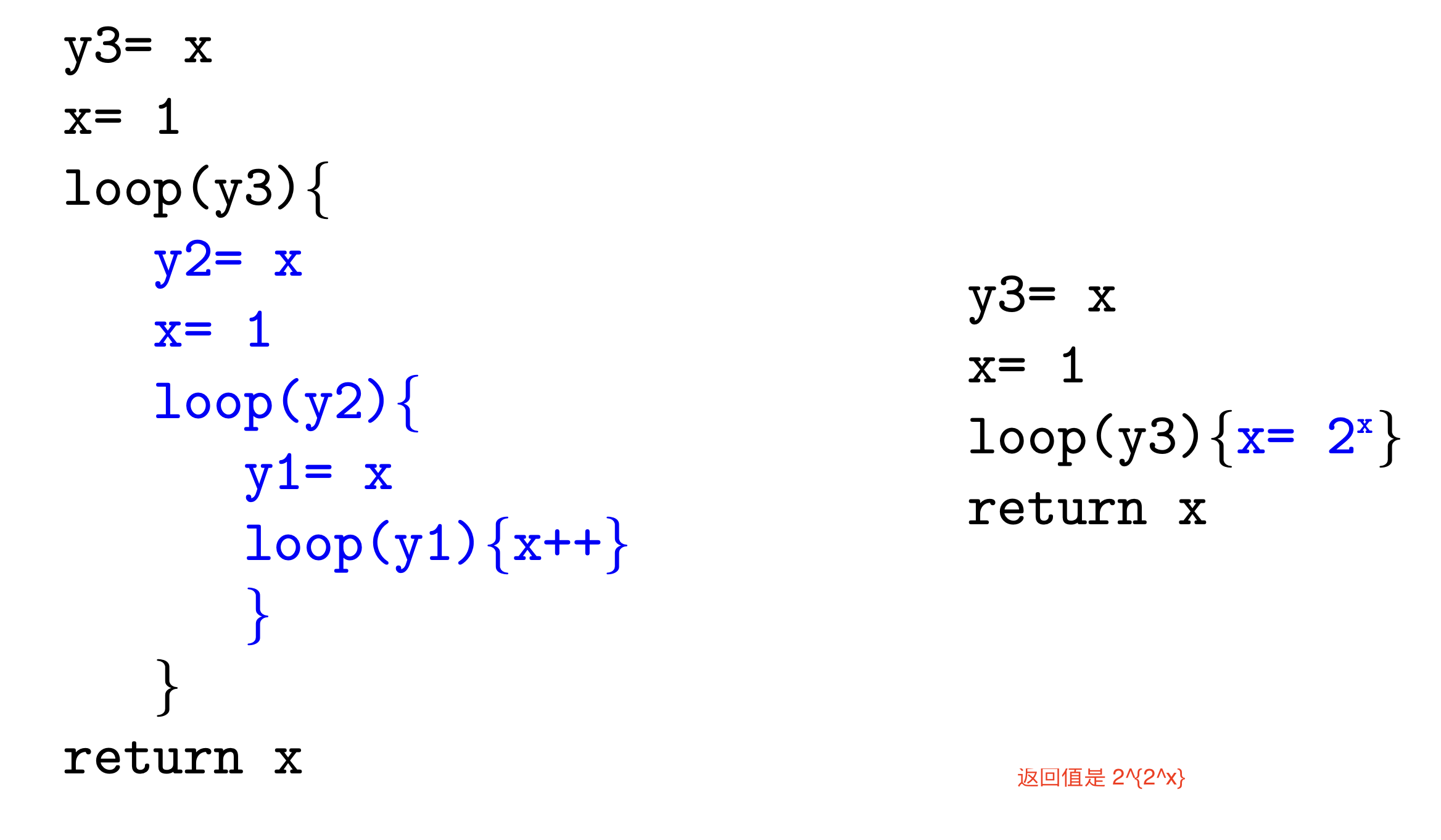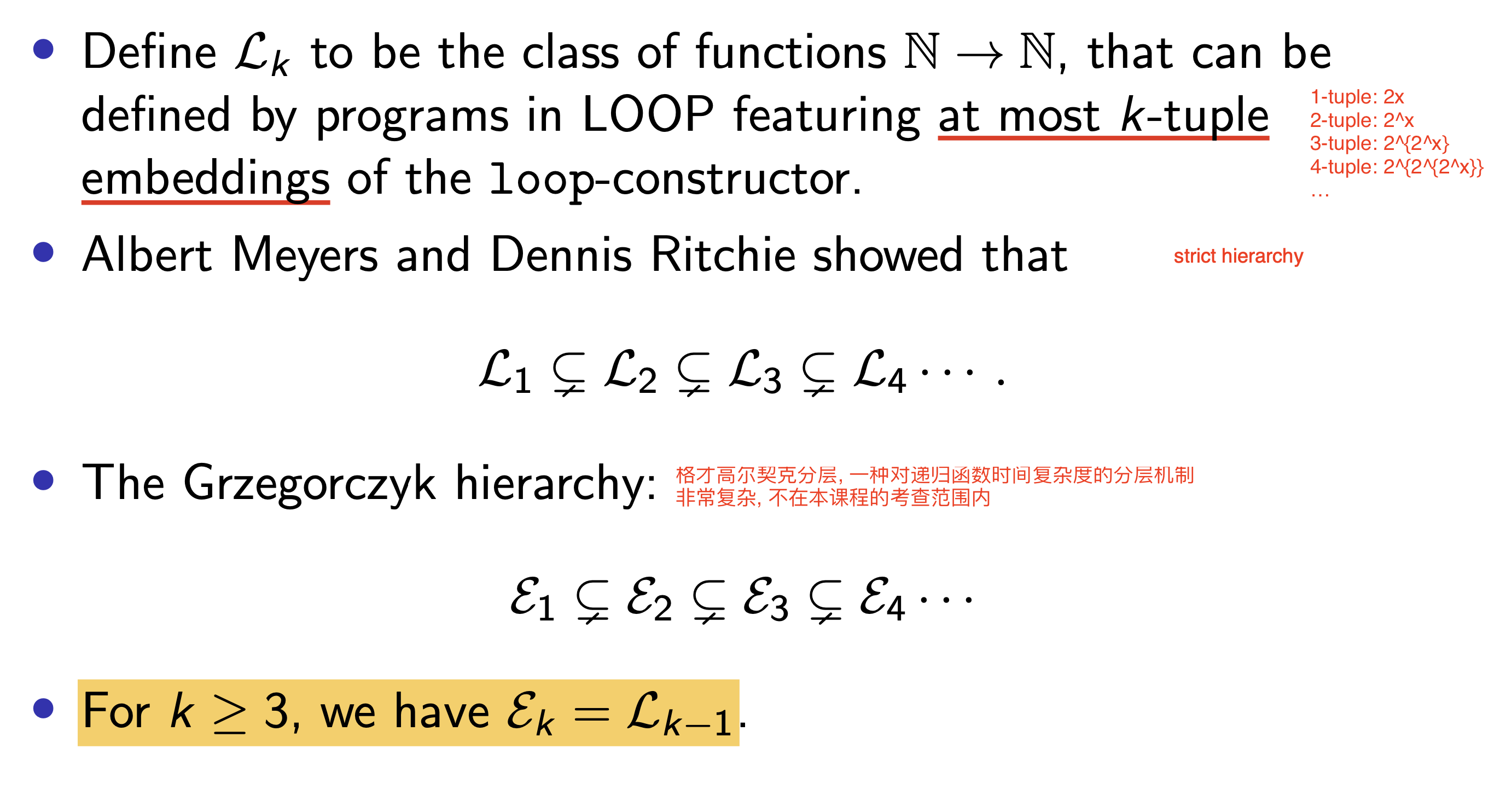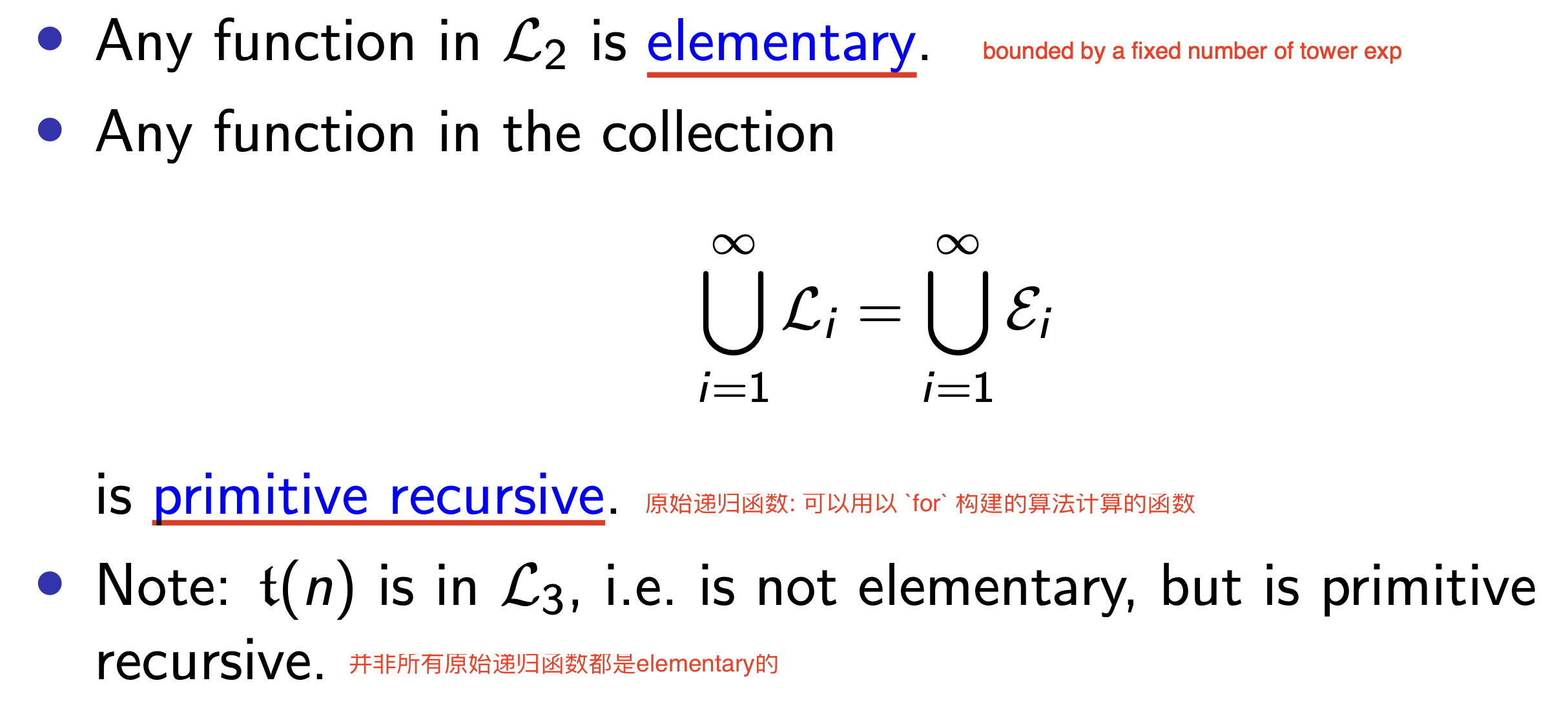### Ackermann 函数

Ackermann 函数是一种 递归定义, 增长速度 更快非原始递归 函数. 它是如下定义的: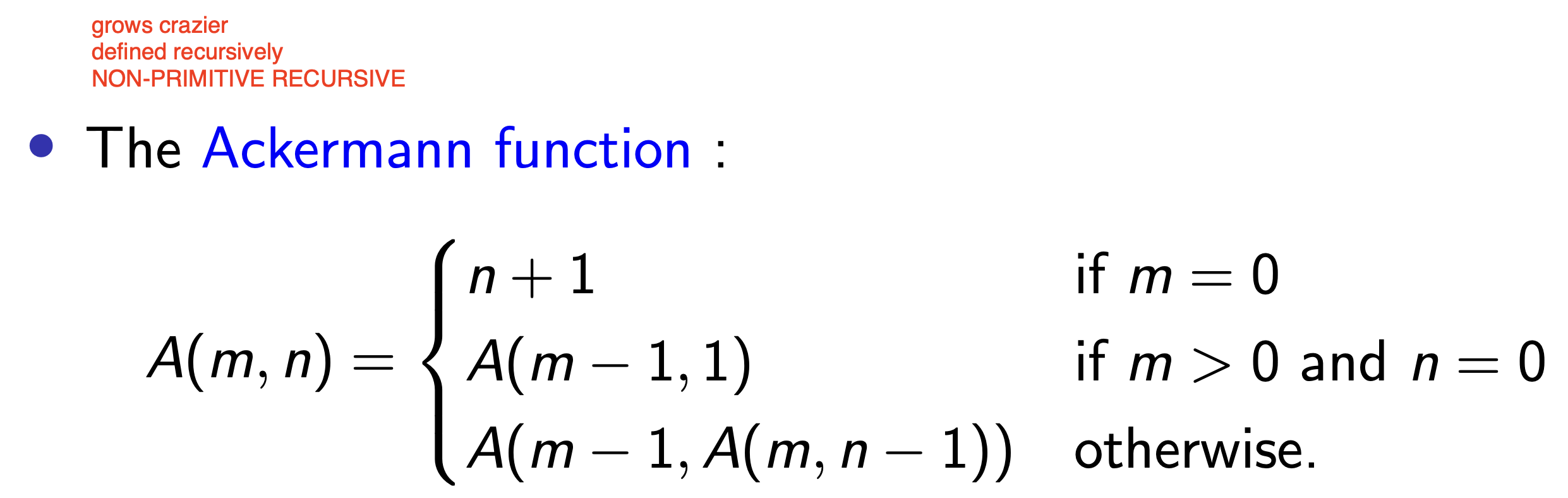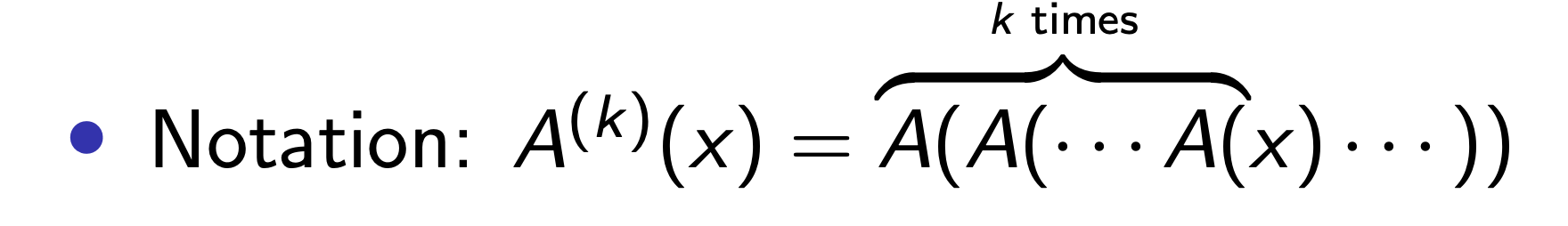Ackermann 函数的反函数记为 $\alpha(n)$. 它接受输入 $A(x)$, 输出 $x$. 换言之:

$\alpha(A(x)) = x.$

## 字符串查找算法

### 问题描述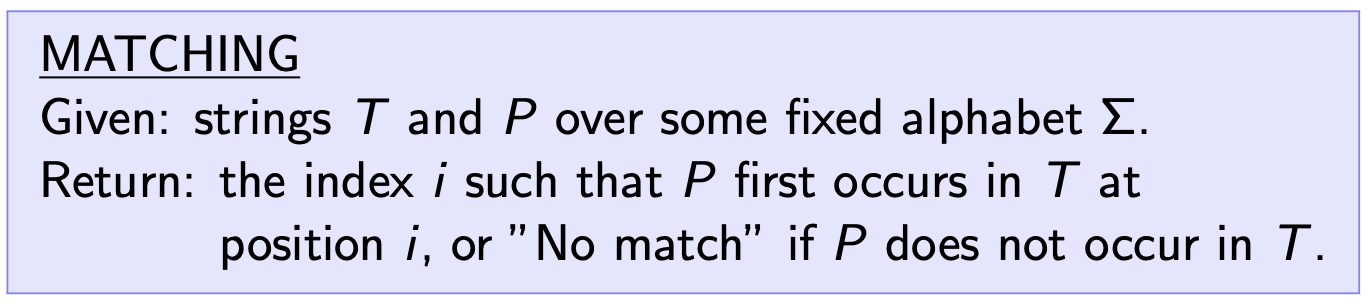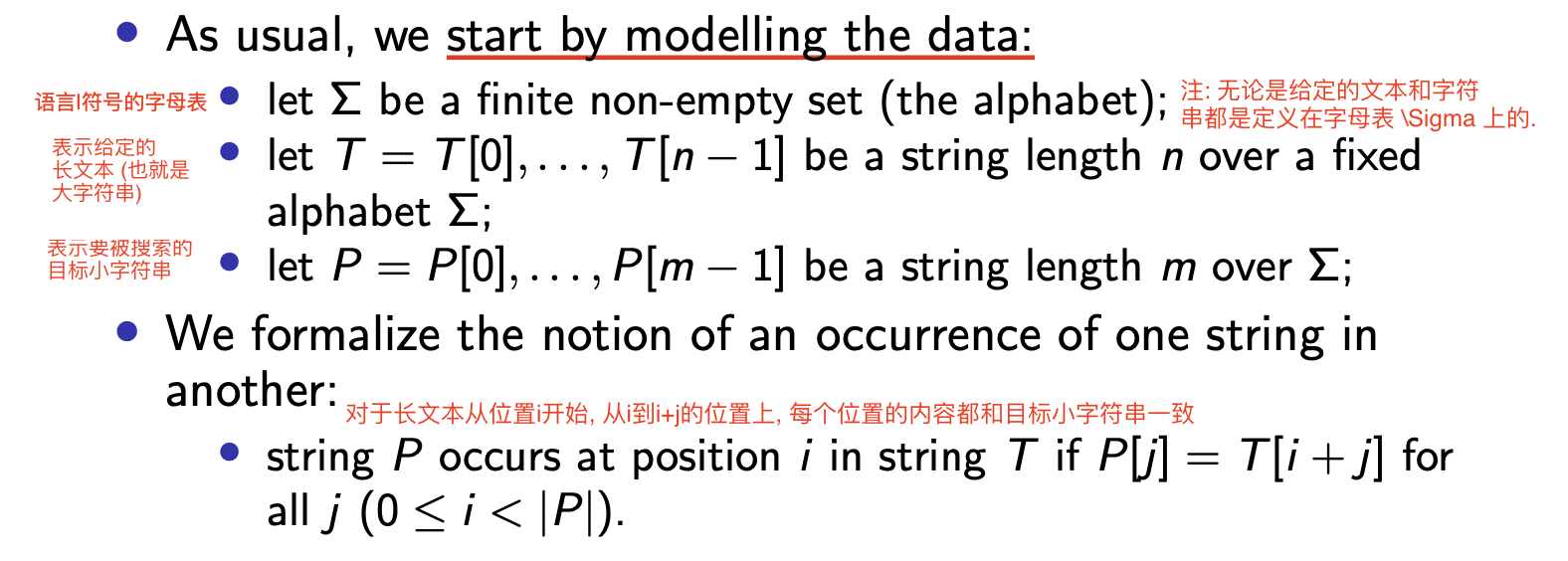### 弱智算法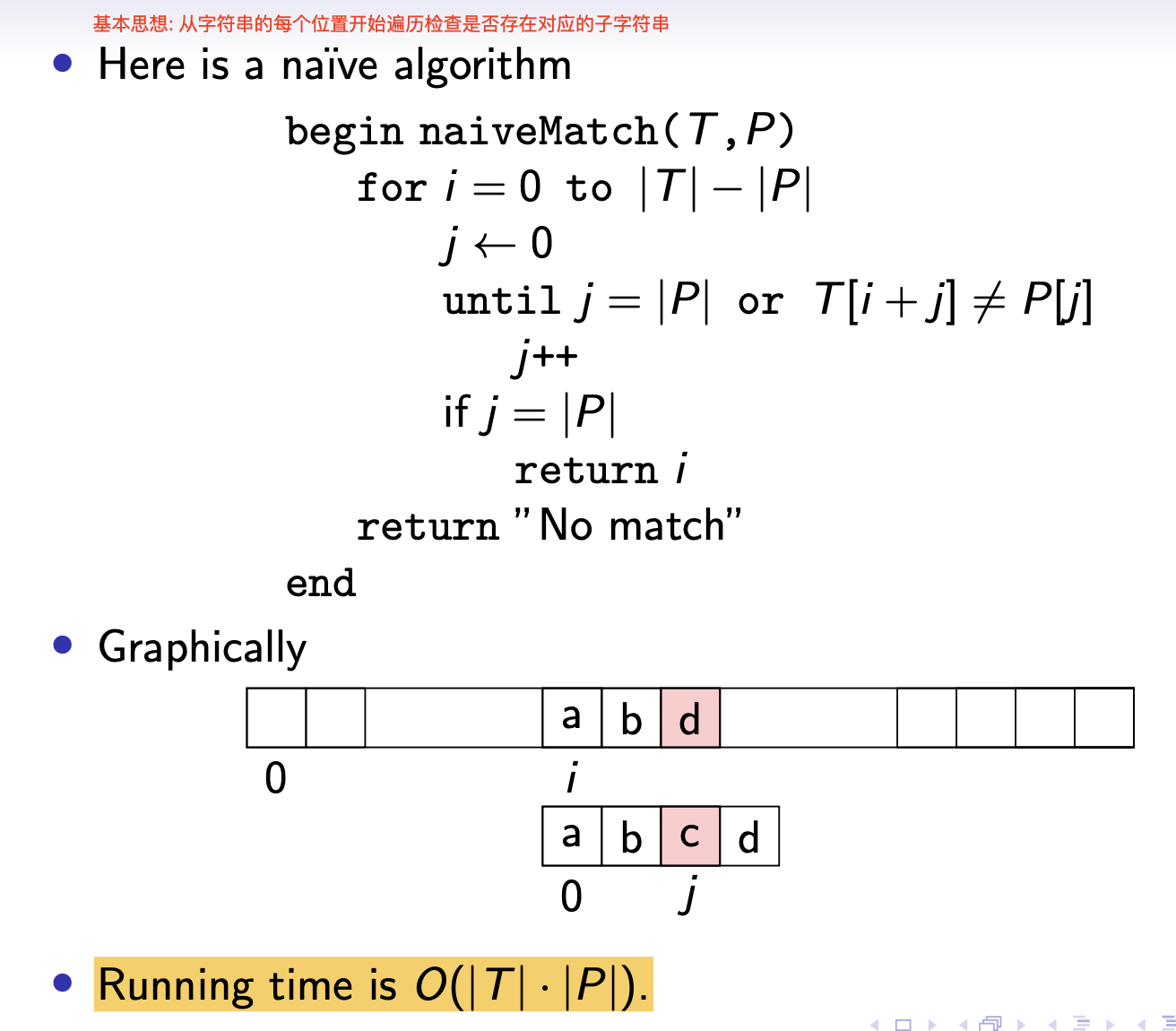### Rabin-Karp 算法

Rabin-Karp 算法的基本思想是: 仿效哈希表中使用数字对字符串编码的方式, 对文本和目标字符串都进行编码, 然后利用编码的数学性质进行检查和搜索. 其具体实现如下:

$P \cdot b^{m-1} + \cdots P[m-1] \cdot b^{0}.$

$T \cdot b^{n-1} + \cdots T[n-1] \cdot b^{0}.$

$T[i, \cdots, i+m-1] = T[i] \cdot b^{m-1} + \cdots + T[i+m-1] \cdot b^{0}.$

1. 目标字符串 $P$ 的 唯一表示
2. 文本 $T$ 中 可能和目标字符串匹配的一个长为 $m$ 的分段的唯一表示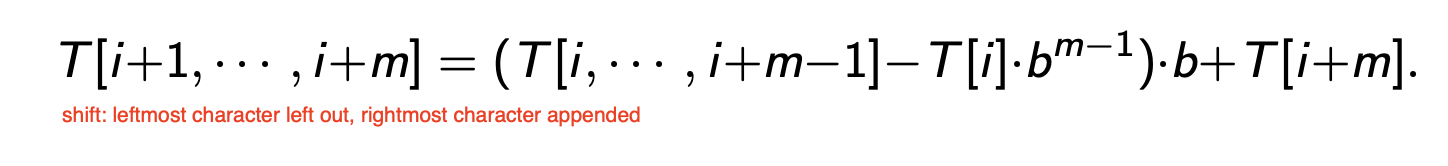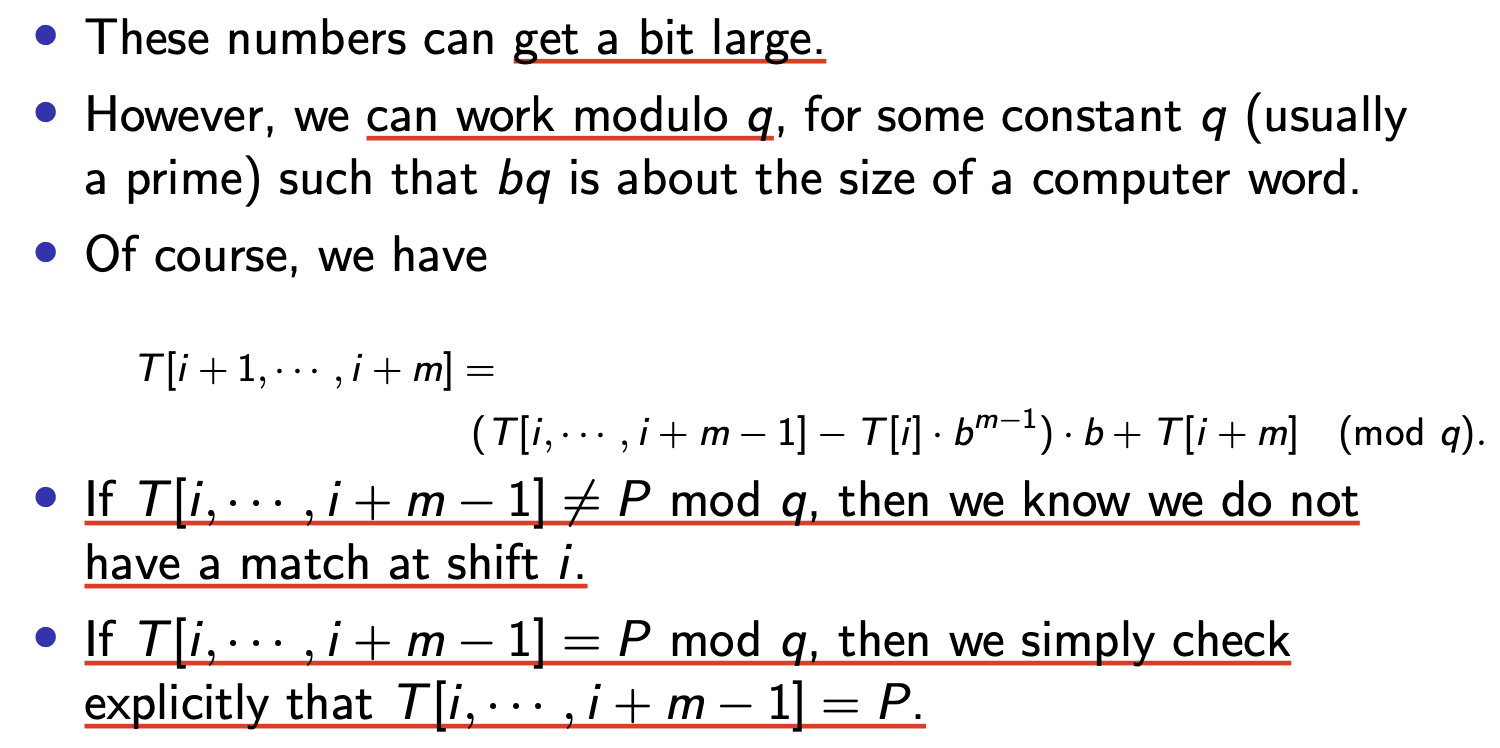$O(m \cdot (\frac{n}{q}))$

$O(n + m + m \cdot (\frac{n}{q}))$

$O(n + m)$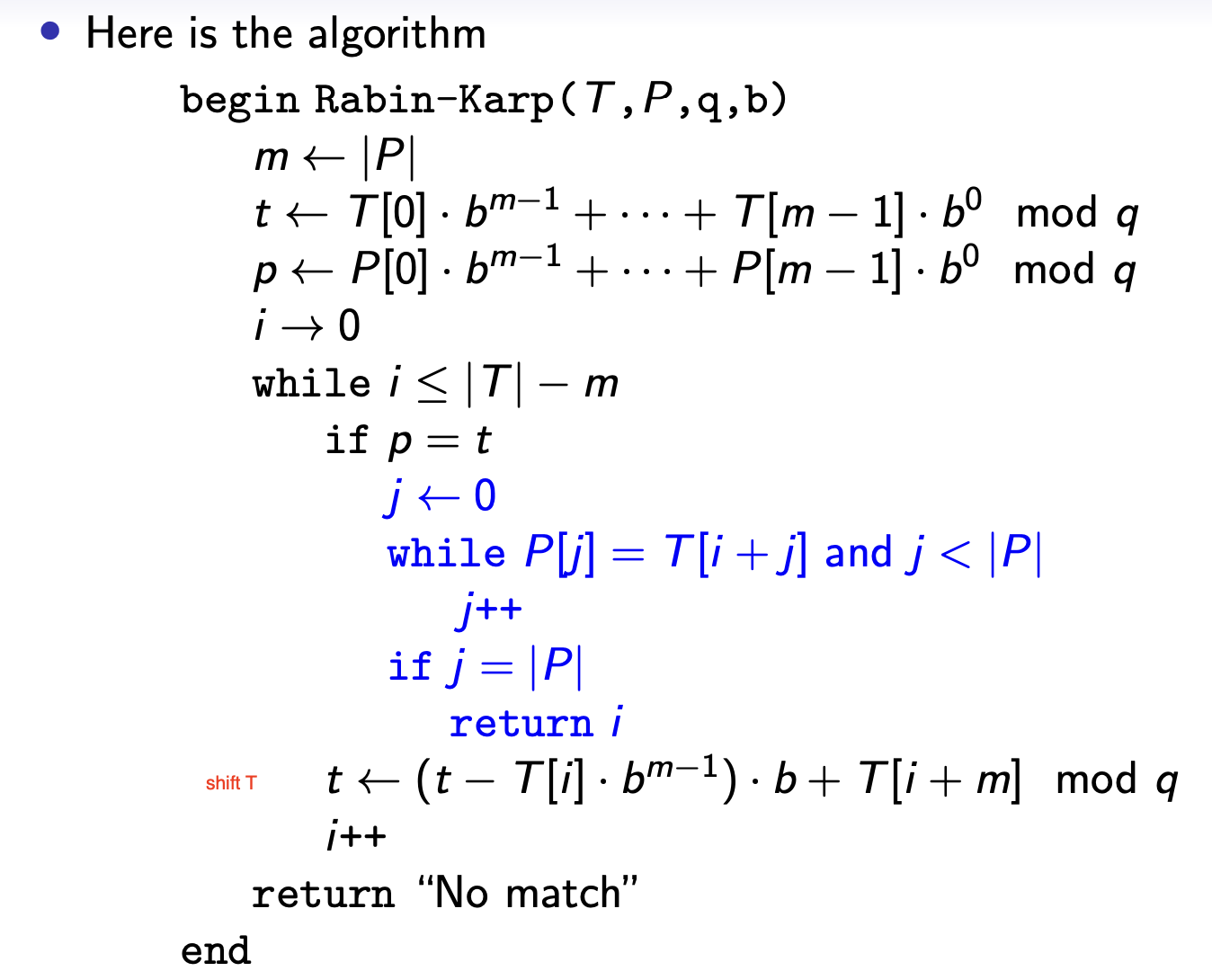### Knoth-Morris-Pratt 算法

KMP 算法要解决的问题仍然是 主串中的字符串匹配问题.

#### KMP 算法中目标字符串的指针的移动规律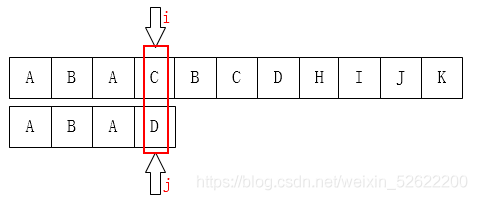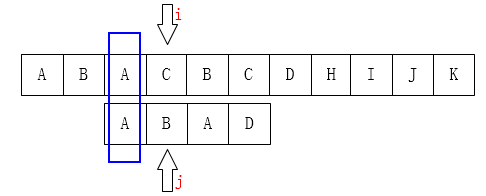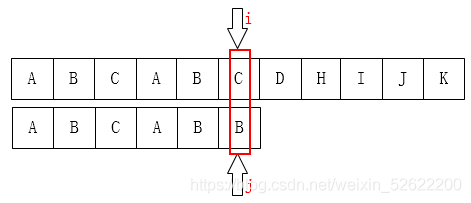1
P[0 : k-1] == P[j-k : j-1]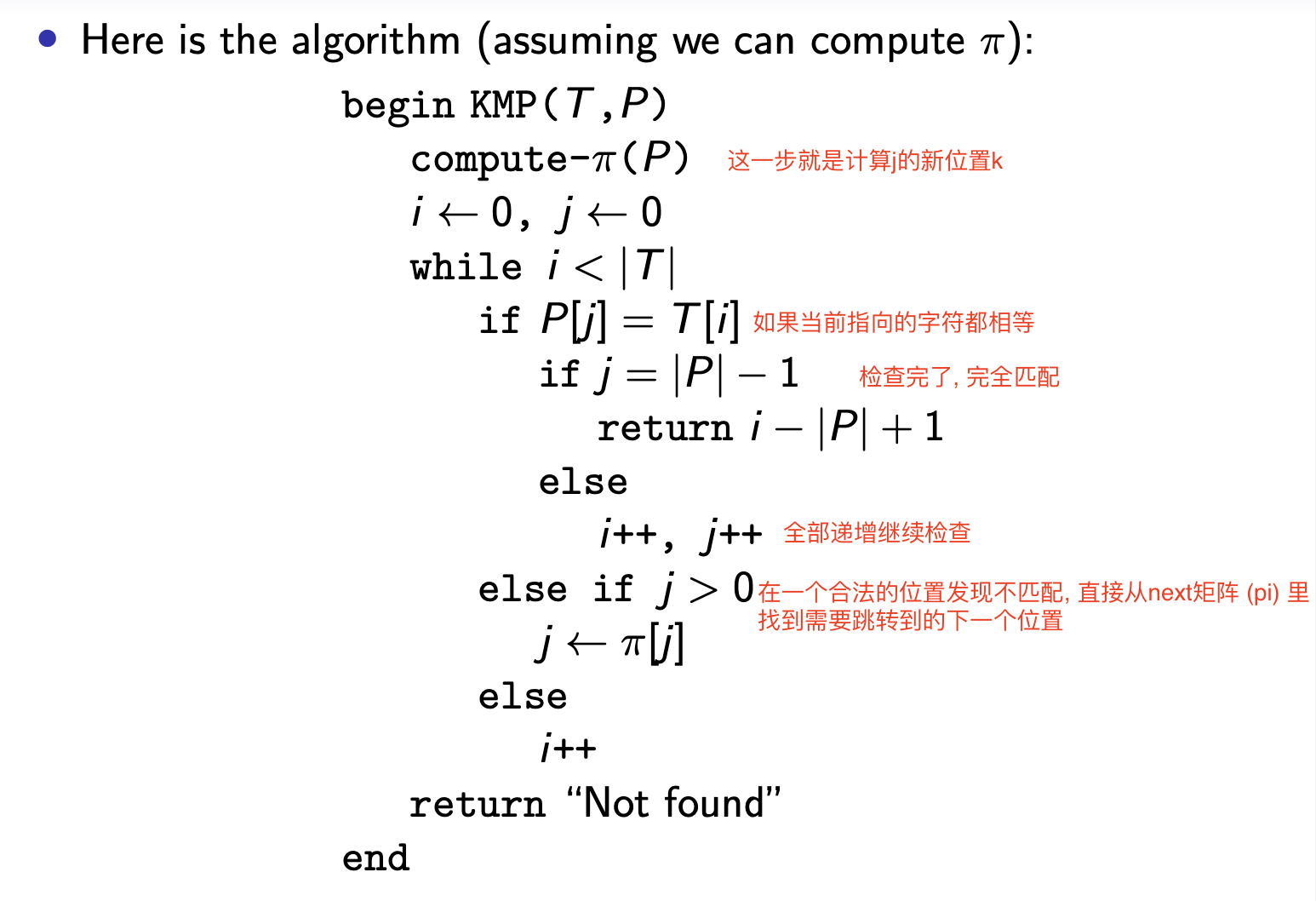#### KMP 算法中对目标指针下一个最适位置的计算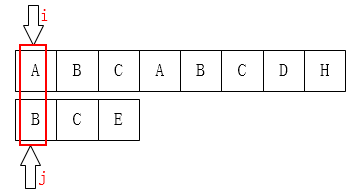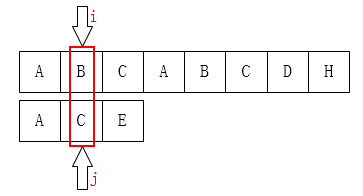$\pi[j+1] = \pi[j] + 1:$

$P[0:k-1] = P[j-k:j-1]$

$P[k] = P[j]$

$P[0:k-1] + P[k] = P[j-k:j-1] + P[j]$

$P[0:k] = P[j-k:j]$

$\pi[j+1] = k+1 = \pi[j] + 1. \blacksquare$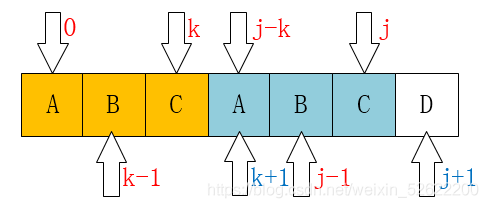1
2
3
4
5
6
7
8
9
10
11
12
13
14
15
def compute-pi():
p = list(p)
next = [-1 for _ in range(len(p))]

j, k = 0, -1

while (j < len(p)-1):
if (k==-1 or p[j] == p[k]):
j = j+1
k = k+1
next[j] = k
else:
k = next[k]

return next


REF: https://blog.csdn.net/u012948161/article/details/126241717

https://blog.csdn.net/weixin_52622200/article/details/110563434